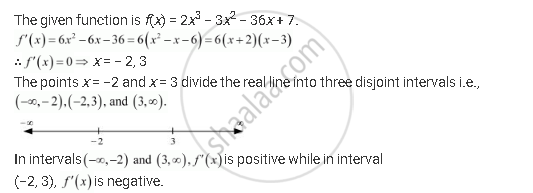Share

# Find the Intervals in Which the Function F Given By F(X) = 2x3 − 3x2 − 36x + 7 is Strictly Increasing and Strictly Decreasing - CBSE (Science) Class 12 - Mathematics

ConceptIncreasing and Decreasing Functions

#### Question

Find the intervals in which the function f given by f(x) = 2x3 − 3x2 − 36x + 7 is

(a) strictly increasing

(b) strictly decreasing

#### Solution

The given function is f(x) = 2x3 − 3x2 − 36x + 7.Hence, the given function (f) is strictly increasing in intervals (-oo, -2) and (3, oo) while function (f) is strictly decreasing in interval (−2, 3).

Is there an error in this question or solution?

#### APPEARS IN

NCERT Solution for Mathematics Textbook for Class 12 (2018 to Current)
Chapter 6: Application of Derivatives
Q: 5 | Page no. 205

#### Video TutorialsVIEW ALL 

Solution Find the Intervals in Which the Function F Given By F(X) = 2x3 − 3x2 − 36x + 7 is Strictly Increasing and Strictly Decreasing Concept: Increasing and Decreasing Functions.
S